Upd：阅读818祭

# 2020.08

（昨天搬宿舍太累了）

i了i了

T4以为看穿了正解逆序对

T3暴力最短路手模一个半小时

T2半个小时调了一个k进制的暴力

11点开T1

%%%%%%%%%%%%

！！

## 2020.8.19

NOI好评

%%%%%%%%%%%%%%%%%%%%%%%

ljh写了个hack数据
Hack了自己还有zyf

xswl

## 2020.8.18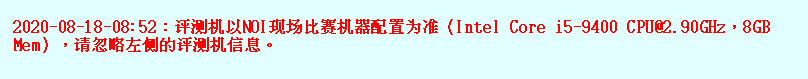i5也可，不赖不赖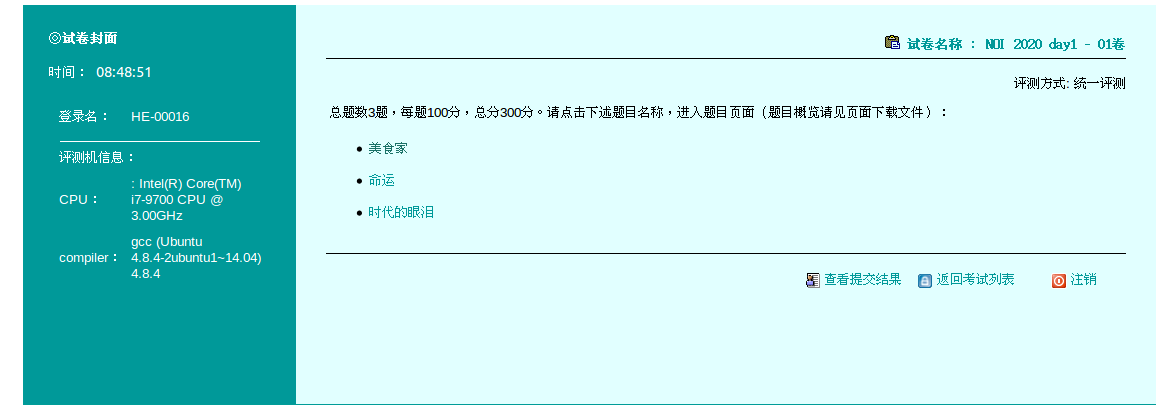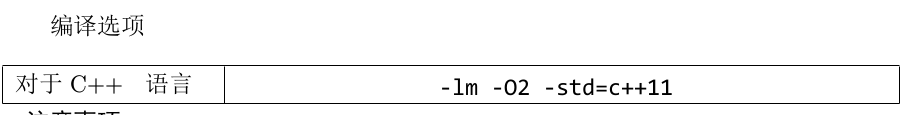$O_2$还有$C++11$泪目泪目

### 818打卡

NOI要来了

$Giao$

## 2020.8.17

$OLG!$

## ff*2

docx命名打错一个字打成了hs，ff锁我喉，我依然没有还手

woc woc woc

T2暴力能A不知道为啥打了个Tarjan结果全T了？？？

## 2020.8.15

### Splay

#include <iostream>
#include <cstring>
#include <cstdio>
#include <algorithm>
using namespace std;

int x = 0, w = 1;
char ch = getchar();
for(; ch > '9' || ch < '0'; ch = getchar()) if(ch == '-') w = -1;
for(; ch >= '0' && ch <= '9'; ch = getchar()) x = x * 10 + ch - '0';
return x * w;
}

const int ss = 100010;
const int inf = 0x7fffffff;

struct node{
int son;
int size, num, val, fa;
}tr[ss << 1];

int root, cnt;

inline void update(int x){
tr[x].size = tr[tr[x].son].size + tr[tr[x].son].size + tr[x].num;
}

inline void connect(int x, int fx, int kx){
if(x) tr[x].fa = fx;
if(fx) tr[fx].son[kx] = x;
}

inline int identify(int x){
return tr[tr[x].fa].son == x;
}

inline void rotate(int x){
int fx = tr[x].fa;
int ffx = tr[fx].fa;
int kx = identify(x);
int kfx = identify(fx);
connect(tr[x].son[kx ^ 1], fx, kx);
connect(fx, x, kx ^ 1);
connect(x, ffx, kfx);
update(fx);
update(x);
}

inline void splay(int x, int goal){
goal = tr[goal].fa;
while(tr[x].fa != goal){
int fx = tr[x].fa;
if(tr[fx].fa == goal) rotate(x);
else if(identify(x) == identify(fx)) rotate(fx), rotate(x);
else rotate(x), rotate(x);
}
if(!goal) root = x;
}

inline void find_val(int val){
int u = root;
while(tr[u].son[val > tr[u].val] && tr[u].val != val)
u = tr[u].son[val > tr[u].val];
splay(u, root);
}

inline int get(int val, int p){
find_val(val);
int u = root;
if(tr[u].val > val && p || tr[u].val < val && !p)
return u;
u = tr[u].son[p];
while(tr[u].son[p ^ 1])
u = tr[u].son[p ^ 1];
return u;
}

inline void insert(int val){
int u = root, fa = 0;
while(u && val != tr[u].val)
fa = u, u = tr[u].son[val > tr[u].val];
if(u) tr[u].num++;
else{
u = ++cnt;
if(fa) tr[fa].son[val > tr[fa].val] = u;
tr[u].val = val;
tr[u].fa = fa;
tr[u].size = tr[u].num = 1;
}
splay(u, root);
}

inline void del(int val){
int get0 = get(val, 0);
int get1 = get(val, 1);
splay(get0, root);
splay(get1, tr[root].son);
int u = tr[get1].son;
if(tr[u].num == 1) tr[get1].son = 0;
else tr[u].num--, tr[u].size--;
tr[get1].size--;
tr[get0].size--;
}

inline int find_kth(int num){
int u = root;
while(1){
if(num > tr[tr[u].son].size + tr[u].num)
num -= tr[tr[u].son].size + tr[u].num, u = tr[u].son;
else if(num <= tr[tr[u].son].size) u = tr[u].son;
else return tr[u].val;
}
}

signed main(){
insert(+inf);
insert(-inf);
while(n--) {
if(op == 1) insert(x);
if(op == 2) del(x);
if(op == 3) find_val(x), printf("%d\n", tr[tr[root].son].size);
if(op == 4) printf("%d\n",find_kth(x + 1));
if(op == 5) printf("%d\n",tr[get(x, 0)].val);
if(op == 6) printf("%d\n",tr[get(x, 1)].val);
}
return 0;
}



### ff吃醋

Update:更新了博客地址

（感觉一下大雨我就降智，中二病犯了）

“我要是12点睡着了，我**的不是人！”

“啊这，昨天晚上睡着了”666

## 2020.8.13

（毕竟码有点长）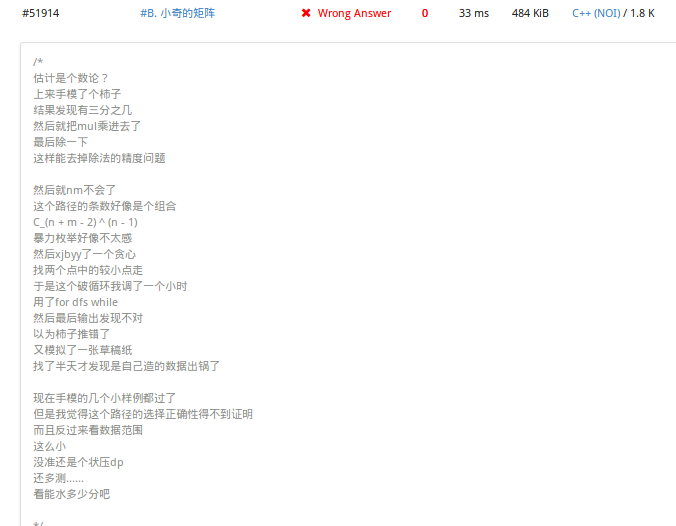T1以为真——笨小猴

T3随便yy了个dfs就10分
T4LCA括号括错了

LCA查错返回dis值竟然是对的？？？

5555

## 2020.8.12

T1怎么说

T2暴力直取30结果只有10？？
T3没看懂题挂了

Jumbo看到我来了直接把游戏退了？？？

“原产地：河北省邯郸市”

## 2020.8.9

（可能比较喜欢图论？）
01规划一共才写了俩

！！！！！（虽然没有留下任何痕迹）

“访问量，评论，兴奋半天，像博主一样”

$QwQ$

01规划还有基环树还没咋练

## 2020.8.8

（省略夸奖词一千字）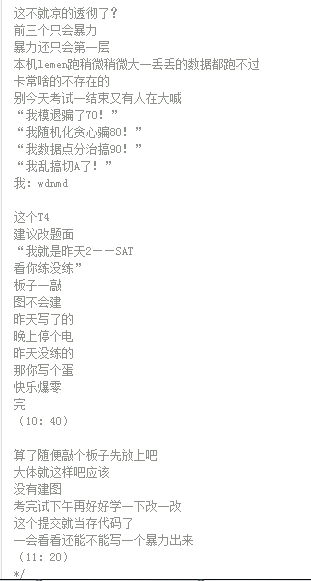（其实都不舍得）

## 2020.8.5

Y（袁）xy学长讲了个T4

i了i了

T4打算今天改完把题解写一下## 2020.8.4

### 阅读520祭

21:02:04

T4一道题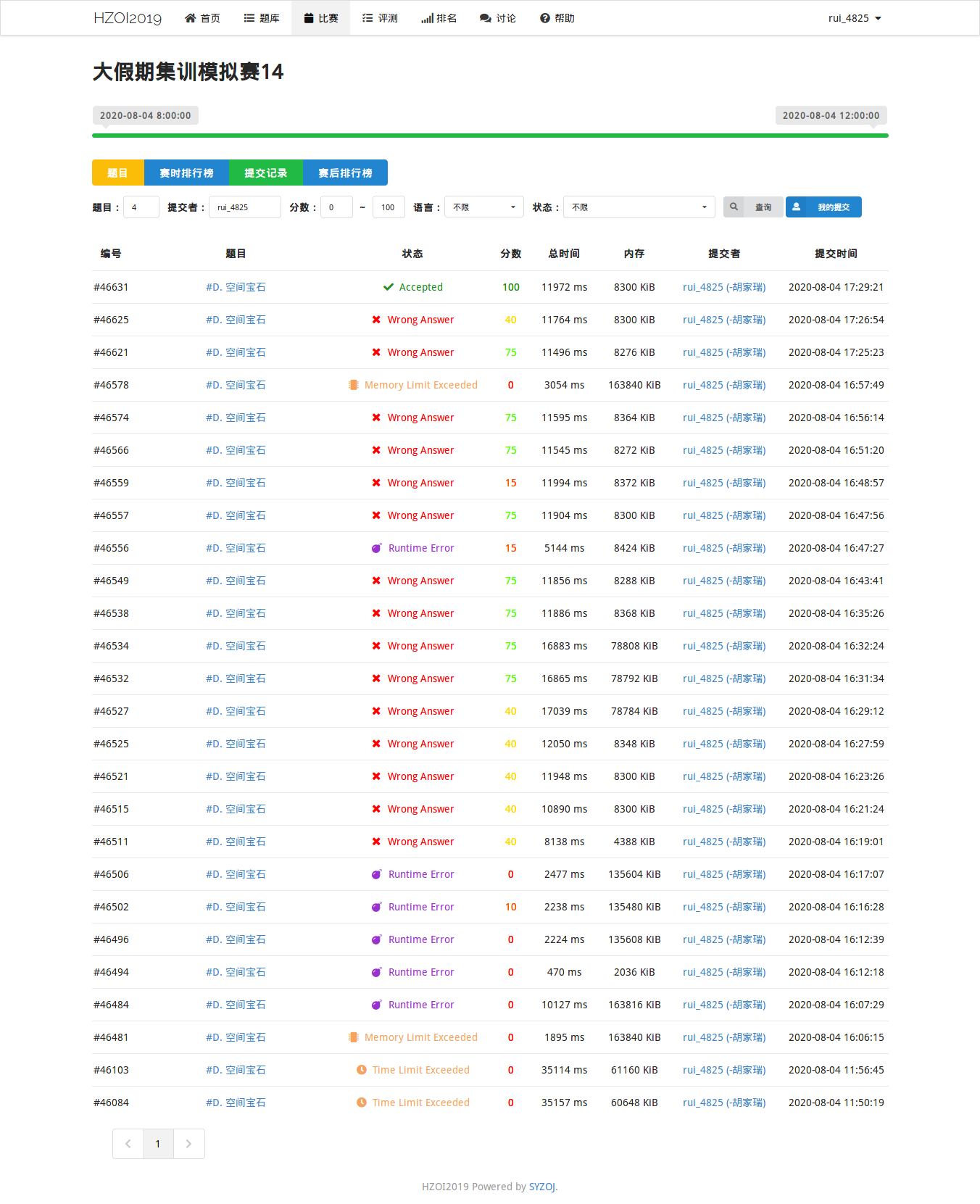T1想正解想了俩小时

mlgb

T2同上

freopen没删

T4暴力挂了

fwfwfwfw

## 2020.8.2

zxk说看到标签就盲猜打了一下就过了

## 2020.8.1

rp-=INF

lzz大声宣布
“这是hjr造的数据！跟我没关系！你们都打他！”

emmmm

T1说是签到题但是卡了两个小时

T3T4都是暴力
T3应该是数论
T4正解应该是线段树
T2dfs不会处理



# 2020.07

## 2020.7.31

T4给的舒坦

yxy对T1yy出来一个式子

（已经上传到ftp2019）
lemen本地测试了近20人的代码

xswl

## 2020.7.29

T1T3真的是，，，，

T3推出来的结论没有归纳

T4挂-1输出qj测试点都比T1T3分多

T4NOIP原题连看都没看

kill -9 -1都没用了

2G内存莫名其妙就爆了

……

（尤其不要诱惑我恰零食）
（关键是咱们都造完了）

xswl

T1思路对了，因为开始思路不清晰

T2同样笔误了一个地方

T3T4没啥兴趣不想写了

## 2020.7.26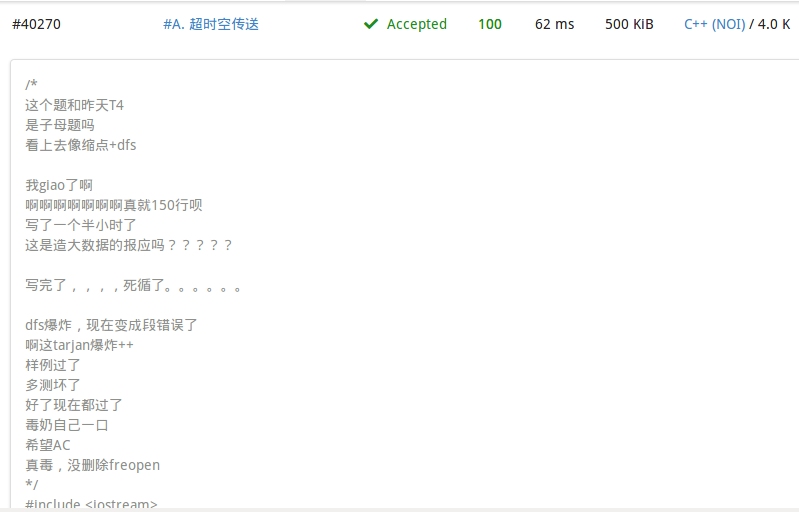wdnmd！！！！！！！

…………

## 2020.7.25

400分满分大多数在100分徘徊

T1T2T3看着都跟二分有关系

T2正解是二分，其他两个多多少少沾点边
（T1暴力+二分比纯暴力多十分）

rp-=INF

## 2020.7.24

！！！！！！！！

T2递归函数有两行写反了

AC——>WA7

wdnmd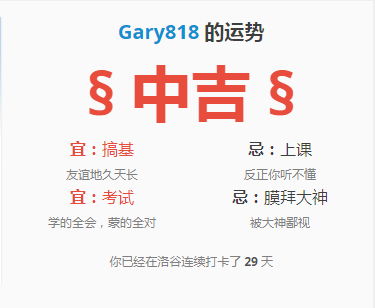emmmmm

xswl

i了i了

## 2020.7.22

T1线段树，先打了暴力没有交

T3 n方暴力没想到
T4 暴枚$LCA$不会统计路径

## 2020.7.21

T3T4除了要合理yy打标出一些规律

## 2020.7.20

### 一周·周记

（小声bb：没有美人一起赏景）

（也不知道为啥今天晚上忽然间这么煽情QaQ）

## 2020.7.19

$Rubyonly$催更，打到博客来写总结

T1一个二分调一个小时，最后玄学判过样例
T2拿到之后随便yy一手

Rubyonly用同样的玄学算法只拿到了30唔哈哈

T3，T4都没啥思路随便码了个暴力交上

$Wilson$定理推不出来找林sir讨论了一波搞懂了

## 2020.7.18

### 数论复习

• 逆元，素数等
• 学习$Miller Rabin$ （判定单个素数）
• 复习$CRT$ & $Lucas$ （中国剩余定理&卢卡斯定理）
• 复习$Catalan$ $number$ （卡特兰数，最近考试有一个蛮像的）

“这大早晨的就喝”
“怕一会犯困”

rp-=INF

ye青回一下，323，#11
T1kruskal，一眼看穿

T3原题没啥好写的

rp大增

nmb这两天正复习图论

12点出结果，泥OI人均300

Luogu交了一页

Xjb想了四五种方法最终爆零

## 2020.7.16

hhy挂了代理上外网，老姚懵逼ing：为啥限制不了黑名单了？

hhy：二楼小机房。

hhy：我也不知。

（以上）

一杯茶，一包烟



## 2020.7.15

### 图论复习

• SPFA/Dij/Floyd
• 差分约束，Tarjan（缩点，割点，割边）
• Kruskal/Prim
• LCA，树剖
• 二分图，拓扑排序

## 2020.7.14

posted @ 2020-07-14 17:54  Gary_818  阅读(950)  评论(130编辑  收藏